Courses

# Rotational Motion MCQ Level - 1

## 40 Questions MCQ Test | Rotational Motion MCQ Level - 1

Description
This mock test of Rotational Motion MCQ Level - 1 for Class 11 helps you for every Class 11 entrance exam. This contains 40 Multiple Choice Questions for Class 11 Rotational Motion MCQ Level - 1 (mcq) to study with solutions a complete question bank. The solved questions answers in this Rotational Motion MCQ Level - 1 quiz give you a good mix of easy questions and tough questions. Class 11 students definitely take this Rotational Motion MCQ Level - 1 exercise for a better result in the exam. You can find other Rotational Motion MCQ Level - 1 extra questions, long questions & short questions for Class 11 on EduRev as well by searching above.
QUESTION: 1

Solution:
QUESTION: 2

Solution:
QUESTION: 3

### A thin uniform rod of mass m and length I is kept on asmooth horizontal surface such that it can move freely.At what distance from centre of rod should a particle ofmass m strike on the rod such that the point P at adistance //3from the end of the rod is instantaneouslyat rest just after the elastic collision?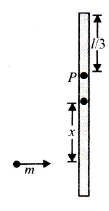Solution:
QUESTION: 4

A yo-yo, arranged as shown, rests on a frictionlesssurface. When a force F is applied to the string, the yo-yo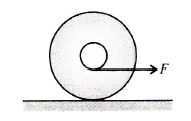Solution:
QUESTION: 5

Velocity of the centre of a small cylinder is v. There isno slipping any where. The velocity of the centre of the larger cylinder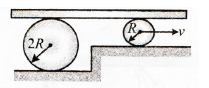Solution:
QUESTION: 6

The figure shows a uniform rod lying along the x-axis.The locus of all the points lying on the x-y plane, aboutwhich the moment of inertia of the rod is same as thatabout O, is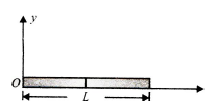Solution:
QUESTION: 7

Find the minimum height of the obstacle so that the sphere can stay in equilibrium.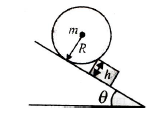Solution:
QUESTION: 8

The spool shown in the figure is placed on a roughhorizontal surface and has inner radius r and outerradius R. The angle θ between the applied force and the horizontal can be varied. The critical angle ( θ ) forwhich the spool does not roll and remains stationaryis given by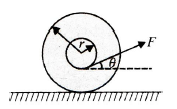Solution:
QUESTION: 9

A small bead of mass m moving with velocity v getsthreaded on a stationary semicircular ring of mass mand radius R kept on a horizontal table. The ring canfreely rotate about its centre. The bead comes to restrelative to the ring. What will be the final angular velocityof the system?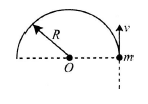Solution:
QUESTION: 10

A sphere is placed rotating with its centre initially at rest in a corner as shown in figures (a) and (b). Coefficient of friction between all surfaces and the sphere is 1/3. Find the ratio of the friction forces fa / fb by ground in situations (a) and (b).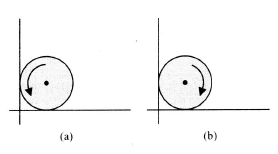Solution:
QUESTION: 11

A block of mass m is attached to a pulley disc of equalmass m radius r by means of a slack string as shown.The pulley is hinged about its centre on a horizontaltable and the block is projected with an initial velocityof 5 m/s. Its velocity when the string becomes tautwill be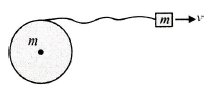Solution:
QUESTION: 12

Four rods of side length / have been hinged to form arhombus. Vertex A is fixed to a rigid support, vertex Cis being moved along the x-axis with constant velocitywas shown in Fig.The rate at which vertex B is nearingthe x-axis at the moment the rhombus is in the form of a square is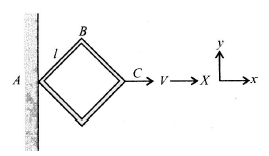Solution:
QUESTION: 13

In the figure shown, the instantaneous speed of end Aof the rod is v’ to the left. The angular velocity of the rodof length L must be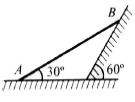Solution:
QUESTION: 14

Two blocks each of mass m and a cylinder C areconnected as shown in Fig. Angular acceleration ofthe cylinder C of radius R is (all strings and pulley areideal):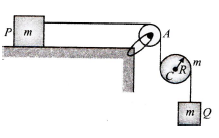Solution:
QUESTION: 15

A uniform rod of mass m and length / is fixed from point A, vhich is at a distance l/4 from one end asshown in the figure. The rod is free to rotate in a verticalplane. The rod is released from the horizontal position.What is the reaction at the hinge, when kinetic energy of the rod is maximum?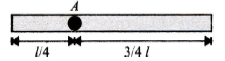Solution:
QUESTION: 16

A uniform pole of length L and mass M is pivoted onthe ground with a frictionless hinge. The pole makesan angle qwith the horizontal. The moment of inertiaof the pole about one end is (1/3) ML2. If it starts fallingfrom the position shown in the accompanying figure,the linear acceleration of the free end of the poleimmediately after release would be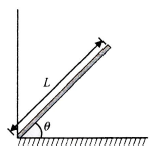Solution:
QUESTION: 17

In the figure shown, a ring A is initially rolling withoutsliding with a velocity v on the horizontal surface of thebody B (of same mass as A). All surfaces are smooth.B has no initial velocity. What will be the maximumheight reached by A on B?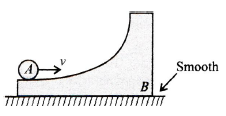Solution:
QUESTION: 18

Two points of a rod move with velocities 3v and vperpendicular to the rod and in the same directionseparated by a distance 'r'. The angular velocity of therod is

Solution:
QUESTION: 19

A sphere is released on a smooth inclined plane fromthe top.When it moves down, its angular momentum is

Solution:
QUESTION: 20

In the figure shown, a ball without sliding on a horizontalsurface. It ascends a curved track up to height h and returns. The value of h is h1 for sufficiently rough curved track to avoid sliding and is h2 for smooth curved track,then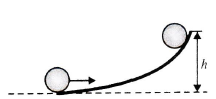Solution:
QUESTION: 21

A ring of radius R rolls without sliding with a constantvelocity. The radius of curvature of the path followed byany particle of the ring at the highest point of its pathwill be

Solution:
QUESTION: 22

A uniform ring of radius R is given a back spin of angularvelocity V0/2R and thrown on a horizontal rough surfacewith velocity of centre to be V0. The velocity of the centreof the ring when it starts pure rolling will be

Solution:
QUESTION: 23

A disc is performing pure rolling on a smooth stationarysurface with constant angular velocity as shown in Fig.At any instant, for the lower most point of the disc,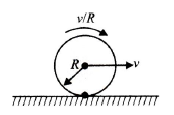Solution:
QUESTION: 24

A particle of mass ‘m’ is rigidly attached at ‘A’ to a ringof mass '3m' and radius ‘r' The system is released fromrest and rolls without sliding. The angular accelerationof ring just after release is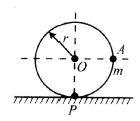Solution:
QUESTION: 25

A solid sphere, a hollow sphere and a disc, all havingthe same mass and radius, are placed at the top of anincline and released. The friction coefficients betweenthe objects and the incline are same and not sufficientto allow pure rolling. The least time will be taken inreaching the bottom by

Solution:
QUESTION: 26

In the previous problem, the smallest kinetic energy atthe bottom of the incline will be achieved by

Solution:
QUESTION: 27

An equilateral prism of mass m rests on a roughhorizontal surface with coefficient of friction μ. Ahorizontal force F is applied on the prism as shown inFig. If the coefficient of friction is sufficiently high so that the prism does not slide before toppling. theminimum force required to topple the prism is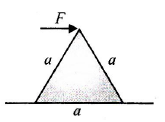Solution:
QUESTION: 28

A unit form disc of radius R lies in the x-y plane, with its centre at origin. Its moment of inertia about z-axis is equal to its moment of inertia about line y = x + c. The value of c will be

Solution:
QUESTION: 29

A mass m is moving at speed v perpendicular to a rodof length a and mass M = 6 m which pivots around africtionless axle running through its centre. It strikesand sticks to the end of the rod. The moment of inertiaof the rod about its centre is Md2/12. Then the angularspeed of the system just after the collision is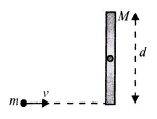Solution:
QUESTION: 30

In the figure shown, a small ball of mass ‘m' can movewithout sliding in a fixed semicircular track of radius R in a vertical plane. It is released from the top. Theresultant force on the ball at the lowest point of thetrack is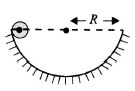Solution:
QUESTION: 31

A uniform rod of mass 15 kg is held stationary with thehelp of a light string as shown in Fig.The tension in thestring is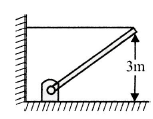Solution:
QUESTION: 32

A solid sphere of mass M and radius R is placed on arough Horizontal surface. It is struck by a horizontalcuestick at a height h above the surface. The value ofh so that the sphere performs pure rolling motionimmediately after it has been struck is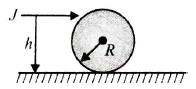Solution:
QUESTION: 33

An object of mass M and radius R is performing purerolling motion on a smooth horizontal surface underthe action of a constant force F as shown in Fig. Theobject may be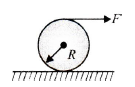Solution:
QUESTION: 34

A cylinder having moment of inertia, which is free torotate about its axis, receives an angular impulse ofJ kg m2/s initially, followed by similar impulse after every 4 s. What is the angular speed of the cylinder 30 safter the initial impulse?

Solution:
QUESTION: 35

A small object of uniform density rolls up a curvedsurface with an initial velocity v. It reaches up to amaximum height of 3v2/4g w.r.t. the initial position. Theobject is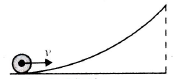Solution:
QUESTION: 36

A solid cylinder of mass M and radius R is resting ona horizontal platform (which is parallel to the x-y plane)with its axis fixed along y-axis and free to rotate aboutits axis. The platform is given a motion in the x-directiongiven by x = A cos (ωt ). There is no slipping betweenthe cylinder and the platform. The maximum torqueacting on the cylinder during its motion is

Solution:
QUESTION: 37

A cubical block of side a is moving with velocity v on ahorizontal smooth plane as shown in Fig. It hits aridge at point O. The angular speed of the block after ithits O is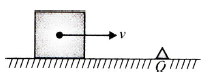Solution:
QUESTION: 38

A solid cylinder is placed on the end of an inclinedplane. It ‘ is found that the plane can be tipped at anangle θ before the cylinder starts to slide. When thecylinder turns on its sides and is allowed to roll, it isfound that the steepest angle at which the cylinder performs pure rolling is Ø . The ratio tan Ø / tan θ is

Solution:
QUESTION: 39

A slender rod of mass m and length L is pivoted abouta horizontal axis through one end and released fromrest at an angle of 30° above the horizontal. The forceexerted by the pivot on the rod at the instant when therod passes through a horizontal position is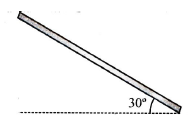Solution:
QUESTION: 40

A slender rod of mass M and length L rests on ahorizontal frictionless surface. The rod is pivoted aboutone of its ends. The impulse of the force exerted onthe rod by the pivot when the rod is struck by a blow ofimpulse J perpendicular to the rod at other end is

Solution: